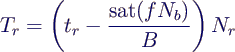# Predator prey systems

• Modeling of predator and prey populations:
• state variables Nb, Nr for number of prey and predator animals
• inflows for births, outflows for deaths
• flows like in population models
• constant birth and death rates
• number of births and deaths proportional to size of population
• interaction between the two species
• F = number of prey hits
• proportional to  Nb and Nr
• directly increases number of prey deaths Tb
• decreases number of predator deaths Tr
• demand B = number of prey animals (per time) needed by a predator to survive
• concrete relations (Lotka-Volterra equations)
•• model PredatorPrey1
•• results
•• typical oscillations
• closed loop in phase diagram ≙ constant of motion
• increase of initial number of prey animals from 50 to 500
•• reduce solver tolerance to 1e-8 !
• Nr rises immediately
• both populations collapse but recover after a long time
• very steep oscillations
• still a closed loop in phase diagram
• very unnatural behaviour!
• problem
• number of catches per predator Fr = F / Nr = f Nb rises with Nb
• idea: limit Fr to a saturation value Fr,max
• Functions defined by graphs:
• saturation curve Fr = sat(f Nb) known only qualitatively
• defined explicitely by a set of  points and linear interpolation
•• implemented with component Mult2GraphConverter
• multiplies its inputs and applies interpolated function to the product
• points defined in catchesPerPredator.txt
• values defined in Modelica as constant array in SystemDynamicsExamples.Resources.PredatorPrey.cpp
• Results of PredatorPrey2B:
• Nb(0) = 50 → same results as before (Fr far from saturation)
• Nb(0) = 500
•• oscillations get stronger!
• reason: Tr becomes negative
•• happened already for PredatorPrey1, but caused limited damage due to constant of motion